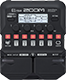# Ride the lighting

Discussion in 'Zoom G1/G1X Four' started by Shad0wl1tvin, Feb 12, 2023.

1. Ride the lightingDevice: Zoom G1 Four
Firmware: 2.00

Name on device: ride the l
Optimized for: Phones/Speaker

Effects chain:fff

Effect: "ZNR" (Dynamics), active - "yes"
"Detect" = GTRIN
"Depth" = 50
"Threshold" = 30
"Decay" = 0

Effect: "MetalWRLD" (Overdrive / Distortion), active - "yes"
"Gain" = 100
"Bass" = 30
"Treble" = 60
"Volume" = 100

Effect: "MS4x12GB" (Cabinet), active - "yes"
"MIC" = ON
"D57: D421" = 0
"Hi" = 65
"Lo" = 30

Effect: "Gt GEQ 7" (Filter), active - "yes"
"100" = 0.0
"200" = 0.0
"400" = -8.5
"800" = -7.0
"1.6k" = -4.0
"3.2k" = 0.0
"6.4k" = 0.0
"VOL" = 100

Effect: "P-P Delay" (Delay), active - "yes"
"Time" = 40
"F.B." = 0
"Mix" = 60
"Tail" = Off

Patch Volume: 100